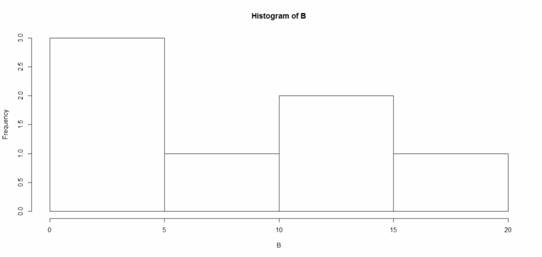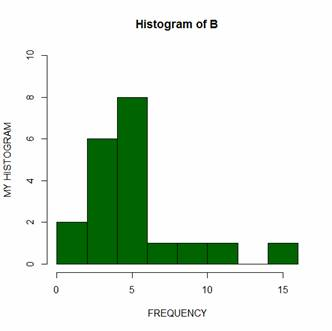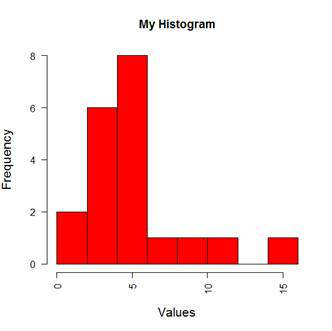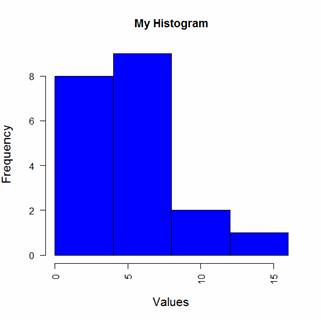# R is Not So Hard! A Tutorial, Part 12: Creating Histograms & Setting Bin WidthsI’m sure you’ve heard that R creates beautiful graphics.

It’s true, and it doesn’t have to be hard to do so.  Let’s start with a simple histogram using the hist() command, which is easy to use, but actually quite sophisticated.

First, we set up a vector of numbers and then we create a histogram.

`B <- c(2, 4, 5, 7, 12, 14, 16)`
`hist(B)`That was easy, but you need more from your histogram. Note that R decided on an appropriate bin width. OK. Now we create a histogram from all the data in an array.

`A <- structure(list(James = c(1L, 3L, 6L, 4L, 9L), Robert = c(2L, 5L, `
`4L, 5L, 12L), David = c(4L, 4L, 6L, 6L, 16L), Anne = c(3L, 5L, `
`6L, 7L, 6L)), .Names = c("James", "Robert", "David", "Anne"), class = "data.frame", row.names = c(NA, -5L))`
`attach(A)`
`A`
`  James Robert David Anne`
`1     1      2     4    3`
`2     3      5     4    5`
`3     6      4     6    6`
`4     4      5     6    7`
`5     9     12    16    6`

The trick is to transform the four variables into a single vector and make a histogram of all elements.

`B <- c(A\$James, A\$Robert, A\$David, A\$Anne)`
`Let’s create a histogram of B in dark green and include axis labels.`
`hist(B, col="darkgreen", ylim=c(0,10), ylab ="MY HISTOGRAM", xlab`
`="FREQUENCY")`However, controlling bin numbers can be problematic. Try setting the number of bins at 6 using the breaks argument.

`hist(B, col = "red", breaks=6, ylim=c(0,8), `
`main="My Histogram", las=2, xlab = "Values", cex.lab = 1.3)`You can see that R has taken the number of bins (6) as indicative only. However, setting up histogram bins as a vector gives you more control over the output. Now we set up the bins as a vector, each bin four units wide, and starting at zero.

`bins<- c(0, 4, 8, 12, 16)`
`hist(B, col = "blue", breaks=bins, ylim=c(0,8), `
`main="My Histogram", las=2, xlab = "Values", cex.lab = 1.3)`Now we have four bins of the right width. That wasn’t so hard! In Part 13 we will look at further plotting techniques in R.

About the Author: David Lillis has taught R to many researchers and statisticians. His company, Sigma Statistics and Research Limited, provides both on-line instruction and face-to-face workshops on R, and coding services in R. David holds a doctorate in applied statistics.Getting Started with R
Kim discusses the use of R statistical software for data manipulation, calculation, and graphical display.

1.JFS says

Again, the table does not read in right using “attach”–maybe it’s due to an R update, or to being on a Mac. But given that the prior one didn’t read in correctly even after jumping through hoops… is there an update needed, or something mac specific?

Here’s what copy-paste gives; it looks like it might be a problem with the tab (or spaces) between the first two columns, which might be handled differently using a mac?:

> A
James Robert David Anne
1 1 2 4 3
2 3 5 4 5
3 6 4 6 6
4 4 5 6 7
5 9 12 16 6
> James Robert David Anne
Error: unexpected symbol in ” James Robert”
> 1 1 2 4 3
Error: unexpected numeric constant in “1 1”
> 2 3 5 4 5
Error: unexpected numeric constant in “2 3”
> 3 6 4 6 6
Error: unexpected numeric constant in “3 6”
> 4 4 5 6 7
Error: unexpected numeric constant in “4 4”
> 5 9 12 16 6
Error: unexpected numeric constant in “5 9”

2.Mayur says

R may not be a good choice for sercah engine optimisation, but they’ve overcome it, I sercahed for R on Google and it was the first result.I’m frustrated with normal stats programs (in some respects, in others I love them), and I’m doing a 3 day R course to see if it can scratch my statistical itch. I’m looking forwards to it, the last time I used a programming language was BASIC in the mid 90s.

Please note that, due to the large number of comments submitted, any questions on problems related to a personal study/project will not be answered. We suggest joining Statistically Speaking, where you have access to a private forum and more resources 24/7.